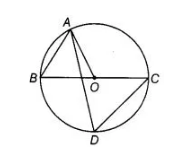# In figure, BC is a diameter of the circle`
Question:

In figure, BC is a diameter of the circle and ∠BAO = 60°. Then, ∠ADC is equal to OA = OB [both are the radius of circle](a) $30^{\circ}$

(d) $45^{\circ}$

(d) $60^{\circ}$

(d) $120^{\circ}$

Solution:

(c) In ΔAOB,

∠OBA = ∠BAO

[angles opposite to equal sides are equal]

∠OBA = 60° [∴ ∠BAO = 60°, given]